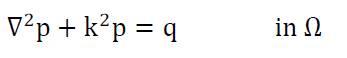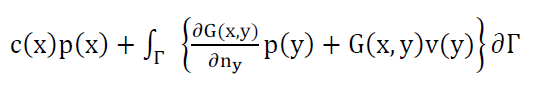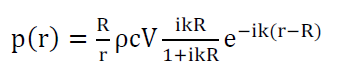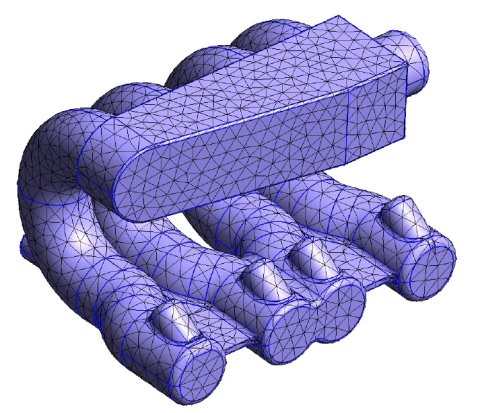# Exterior acoustics with Boundary Element Method

The boundary element method (shortly BEM) is a well-established numerical method which is known within the Academia since the Seventies for the solution of boundary integral equations. It has been proposed to solve a wide range of engineering problems, ranging from structural mechanics up to fluid mechanics, and particularly exterior acoustics (detailed here).

The picture schematically shows a typical exterior acoustic problem. A closed and vibrating body, bounded by a surface Γ, is embedded in an unbounded domain Ω where the pressure wave can propagate. The aim is to predict the pressure and the velocity field on Γ and, potentially, in any point of Ω.

## Challenges:

### A brief summary of the boundary element method (BEM) in acoustics

The Helmoltz equation is the basis of linear acoustics. It can be used to model the propagation of harmonic pressure waves and it can be written as:BEM helmotlz equation (1)

where p is the complex pressure amplitude, q represents the volume sources and k is the wave number. In this work we imagine that the domain Ω is unbounded and that appropriate boundary conditions (Dirichlet, Neuman and Robin) are set to the boundary Γ.

Equation (1) can be numerically solved by means of the FEM, which inevitably requires to introduce a non-physical cut in the unbounded domain Ω, with a consequent violation of the Sommerfeld’s radiation condition at infinity. This means that the numerical model could suffer from undesired spurious wave scattering, therefore producing wrong results.

In literature we can also find an integral version of the Helmoltz equation, which is the starting point for the BEM. Specifically, for exterior problems it is:BEM helmotlz equation integral version(2)

## Solution:

### The pulsating sphere as first benchmark

One of the most used benchmarks in acoustics is the pulsating sphere in the unbounded domain. For this problem also a closed solution is available (see equation (3)) and therefore it is often used to prove the goodness of a specific solver or technique.

The pressure level at a distance r from the sphere center of radius R can be computed as:BEM pulsating sphere equation(3)

BEM pressure level graph

The pressure level on the sphere surface obtained at different frequencies. The exact solution (red) is compared with the numerical solution obtained with (green) and without (blue) the CHIEF technique.

## Results:

###Manifold surface mesh CADThe surface mesh of the manifold. All the corners and sharp edges in the original CAD model have been filled in order to have a smooth surface: in this case the c(x) term in equation (2) can be always set to 0.5.BEM Manifold acoustic simulation

The acoustic pressure expressed in [dB] estimated around the manifold when the vibrating frequency of the manifold is 100 [Hz].